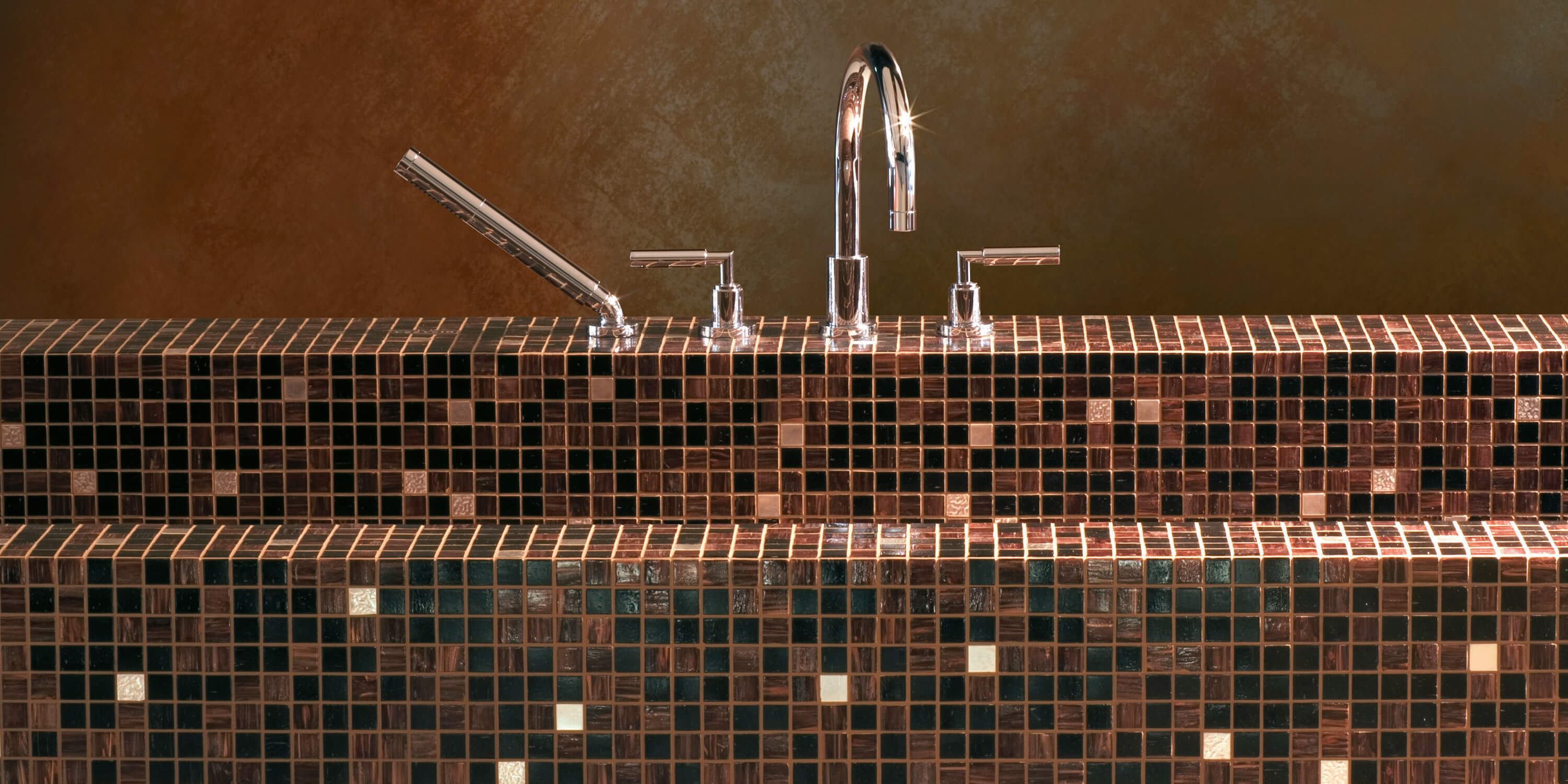### Filter

Content: 1.03 Square meter (€347.70* / 1 Square meter)

€347.70/sqm

Content: 1.03 Square meter (€347.70* / 1 Square meter)

€347.70/sqm

Content: 1.03 Square meter (€347.70* / 1 Square meter)

€347.70/sqm

Content: 1.03 Square meter (€347.70* / 1 Square meter)

€347.70/sqm

Content: 1.03 Square meter (€347.70* / 1 Square meter)

€347.70/sqm

Content: 1.03 Square meter (€347.70* / 1 Square meter)

€347.70/sqm

Content: 1.03 Square meter (€347.70* / 1 Square meter)

€347.70/sqm

Content: 1.03 Square meter (€347.70* / 1 Square meter)

€347.70/sqm

Content: 1.03 Square meter (€347.70* / 1 Square meter)

€347.70/sqm

Content: 1.03 Square meter (€347.70* / 1 Square meter)

€347.70/sqm

Content: 1.03 Square meter (€347.70* / 1 Square meter)

€347.70/sqm

Content: 1.03 Square meter (€347.70* / 1 Square meter)

€347.70/sqm

Content: 1.03 Square meter (€347.70* / 1 Square meter)

€347.70/sqm

Content: 1.03 Square meter (€347.70* / 1 Square meter)

€347.70/sqm

Content: 1.03 Square meter (€347.70* / 1 Square meter)

€347.70/sqm

Content: 1.03 Square meter (€347.70* / 1 Square meter)

€347.70/sqm

Content: 1.03 Square meter (€347.70* / 1 Square meter)

€347.70/sqm

Content: 1.03 Square meter (€347.70* / 1 Square meter)

€347.70/sqm

Content: 1.03 Square meter (€359.90* / 1 Square meter)

€359.90/sqm

Content: 1.03 Square meter (€359.90* / 1 Square meter)

€359.90/sqm

Content: 1.03 Square meter (€347.70* / 1 Square meter)

€347.70/sqm

Content: 1.03 Square meter (€347.70* / 1 Square meter)

€347.70/sqm

Content: 1.03 Square meter (€347.70* / 1 Square meter)

€347.70/sqm

Content: 1.03 Square meter (€347.70* / 1 Square meter)

€347.70/sqm

Content: 1.03 Square meter (€4,300.50* / 1 Square meter)

€4,300.50/sqm

Content: 1.03 Square meter (€4,300.50* / 1 Square meter)

€4,300.50/sqm

Content: 1.03 Square meter (€4,300.50* / 1 Square meter)

€4,300.50/sqm

Content: 1.03 Square meter (€4,300.50* / 1 Square meter)

€4,300.50/sqm

Content: 1.03 Square meter (€342.82* / 1 Square meter)

€342.82/sqm

Content: 1.03 Square meter (€342.82* / 1 Square meter)

€342.82/sqm

Content: 1.03 Square meter (€342.82* / 1 Square meter)

€342.82/sqm

Content: 1.03 Square meter (€342.82* / 1 Square meter)

€342.82/sqm

Content: 1.03 Square meter (€342.82* / 1 Square meter)

€342.82/sqm

Content: 1.03 Square meter (€342.82* / 1 Square meter)

€342.82/sqm

Content: 1.03 Square meter (€342.82* / 1 Square meter)

€342.82/sqm

Content: 1.03 Square meter (€342.82* / 1 Square meter)

€342.82/sqm

Content: 1.03 Square meter (€342.82* / 1 Square meter)

€342.82/sqm

Content: 1.03 Square meter (€342.82* / 1 Square meter)

€342.82/sqm

Content: 1.03 Square meter (€342.82* / 1 Square meter)

€342.82/sqm

Content: 1.03 Square meter (€342.82* / 1 Square meter)

€342.82/sqm

Content: 1.03 Square meter (€342.82* / 1 Square meter)

€342.82/sqm

Content: 1.03 Square meter (€342.82* / 1 Square meter)

€342.82/sqm

Content: 1.03 Square meter (€342.82* / 1 Square meter)

€342.82/sqm

Content: 1.03 Square meter (€342.82* / 1 Square meter)

€342.82/sqm

Content: 1.03 Square meter (€342.82* / 1 Square meter)

€342.82/sqm

Content: 1.03 Square meter (€342.82* / 1 Square meter)

€342.82/sqm

Content: 1.03 Square meter (€342.82* / 1 Square meter)

€342.82/sqm

Content: 1.03 Square meter (€342.82* / 1 Square meter)

€342.82/sqm

Content: 1.03 Square meter (€342.82* / 1 Square meter)

€342.82/sqm

Content: 1.03 Square meter (€342.82* / 1 Square meter)

€342.82/sqm

Content: 1.03 Square meter (€342.82* / 1 Square meter)

€342.82/sqm

Content: 1.03 Square meter (€342.82* / 1 Square meter)

€342.82/sqm

Content: 1.03 Square meter (€4,832.42* / 1 Square meter)

€4,832.42/sqm

Content: 1.03 Square meter (€4,832.42* / 1 Square meter)

€4,832.42/sqm

Content: 1.03 Square meter (€4,832.42* / 1 Square meter)

€4,832.42/sqm

Content: 1.03 Square meter (€4,832.42* / 1 Square meter)

€4,832.42/sqm

Content: 1.03 Square meter (€1,050.42* / 1 Square meter)

€1,050.42/sqm

Content: 1.03 Square meter (€1,050.42* / 1 Square meter)

€1,050.42/sqm

Content: 1.03 Square meter (€1,050.42* / 1 Square meter)

€1,050.42/sqm

Content: 1.03 Square meter (€1,050.42* / 1 Square meter)

€1,050.42/sqm

Content: 1.03 Square meter (€1,050.42* / 1 Square meter)

€1,050.42/sqm

Content: 1.03 Square meter (€1,050.42* / 1 Square meter)

€1,050.42/sqm

Content: 1.03 Square meter (€1,050.42* / 1 Square meter)

€1,050.42/sqm

Content: 1.03 Square meter (€1,050.42* / 1 Square meter)

€1,050.42/sqm

Content: 2.07 Square meter (€118.34* / 1 Square meter)

€118.34/sqm

Content: 2.07 Square meter (€118.34* / 1 Square meter)

€118.34/sqm

Content: 2.07 Square meter (€118.34* / 1 Square meter)

€118.34/sqm

Content: 2.07 Square meter (€118.34* / 1 Square meter)

€118.34/sqm

Content: 2.07 Square meter (€118.34* / 1 Square meter)

€118.34/sqm

Content: 2.07 Square meter (€118.34* / 1 Square meter)

€118.34/sqm

Content: 2.07 Square meter (€118.34* / 1 Square meter)

€118.34/sqm

Content: 2.07 Square meter (€118.34* / 1 Square meter)

€118.34/sqm

Content: 2.07 Square meter (€118.34* / 1 Square meter)

€118.34/sqm

Content: 2.07 Square meter (€118.34* / 1 Square meter)

€118.34/sqm

Content: 2.07 Square meter (€118.34* / 1 Square meter)

€118.34/sqm

Content: 2.07 Square meter (€118.34* / 1 Square meter)

€118.34/sqm

Content: 2.07 Square meter (€118.34* / 1 Square meter)

€118.34/sqm

Content: 2.07 Square meter (€118.34* / 1 Square meter)

€118.34/sqm

Content: 2.07 Square meter (€118.34* / 1 Square meter)

€118.34/sqm

Content: 2.07 Square meter (€118.34* / 1 Square meter)

€118.34/sqm

Content: 2.07 Square meter (€115.90* / 1 Square meter)

€115.90/sqm

Content: 2.07 Square meter (€115.90* / 1 Square meter)

€115.90/sqm

Content: 2.07 Square meter (€176.90* / 1 Square meter)

€176.90/sqm

Content: 2.07 Square meter (€176.90* / 1 Square meter)

€176.90/sqm